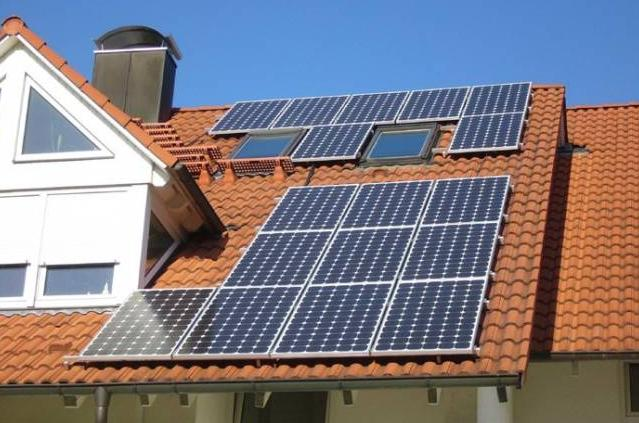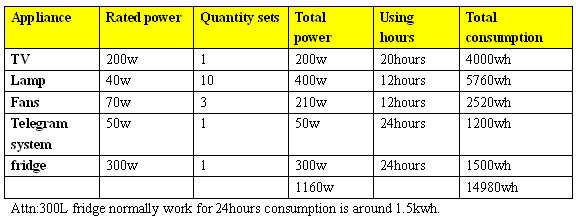How to make off-gird solar system solution

2013-05-17

Rate this item

(1 Vote)How to make an suitable off-grid solar system solution?

step 1.collecting basic datas based on simple questions.

What kind of home  appliance and quantity do you want to load?

How much power of each appliance?

How many hours do you want to run each appliance by solar power everyday?

How many backup days you want to run by solar system?

Step 2. work out total power consumption.

The more exact&complete datas you collect,the more suitable solar system solution it will be.

For example:Step 3. Calculating out  battery solution

Battery=14980wh*1.3(power loss in process)/12v=1622ah

Battery solution according to calculation: 200Ah battery  8pcs

Step 4. Calculating out solar panel solution

Solar panel=(14980wh*1.3*1.3)/8h(sun shine time)=3164w

Solar panel solution according to calculation: 200w solar panel/16pcs

Step 5. Calculating out solar Inverter size

Inverter: Choose  according to total power.Total power is 1160w,suggest using 1500w inverter to leave some

space,reduce the inverter pressure.

Considering solar inverer voltage 24V for 1500W in priority.

Step 6. Calculating out conteroller size

Controller: voltage 24v is fixed according to solar Inverter.

Controller Ampere=3200w solar panel/24V=133.3A

Controller reference solution: 24V150A

Max connecting solar panel:3600w

step 8. consider about solar panel&solar battery connection

1.Battery voltage should connect into 24V,2pcs 12V battery connect in series.

2.Solar panel voltage should connect into 36V,2pcs 18V solar panel connect in series.

Basic theory tips

1.controller,battery,inverter voltage is the same voltage.

2.Best charging connection way is solar panel voltage is higher 1.5 time than battery.

3.Different connection way could change the solar panel and battery voltage.

4.Max connection solar panel watts power=controller ampere*controller voltage

5.Normally the inverter  power should be beyond the total appliance power,

it will reduce the inverter pressure and pro-long inverter life.

6.Solar panel decide charging time for battery,battery decide how many hours could

support appliance.

Hits：    【Print
Welcome ESOLAR!